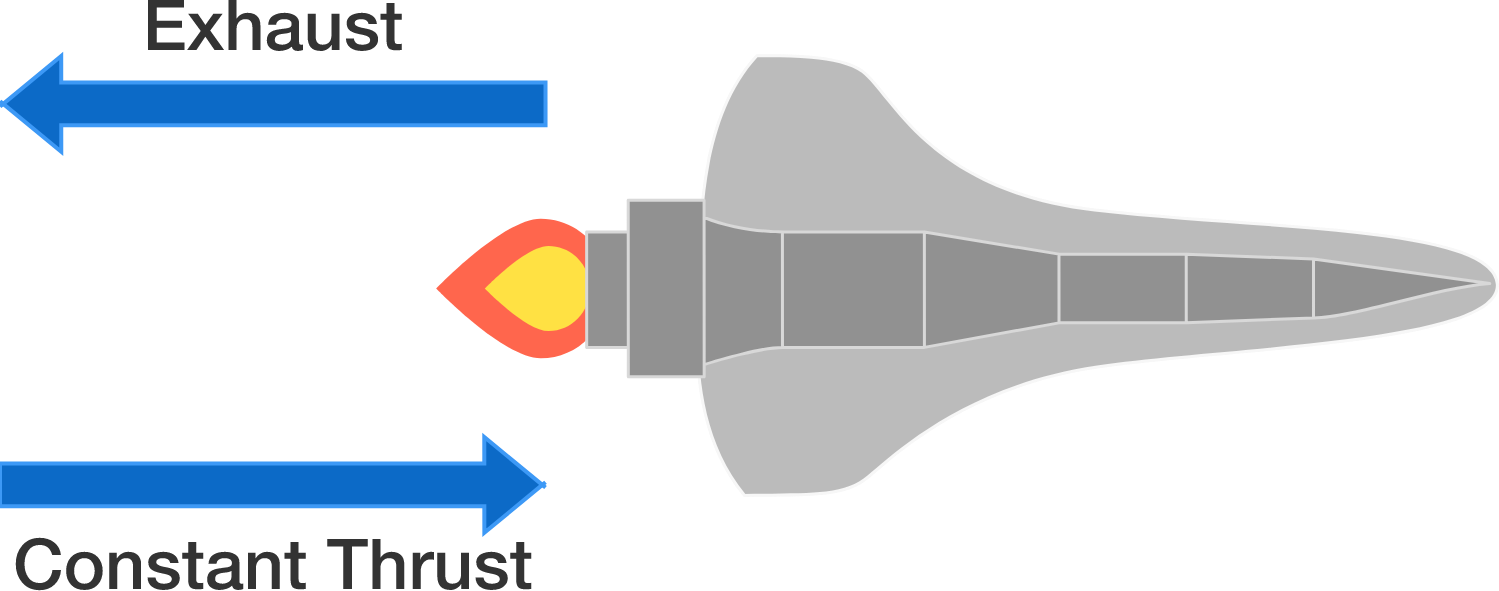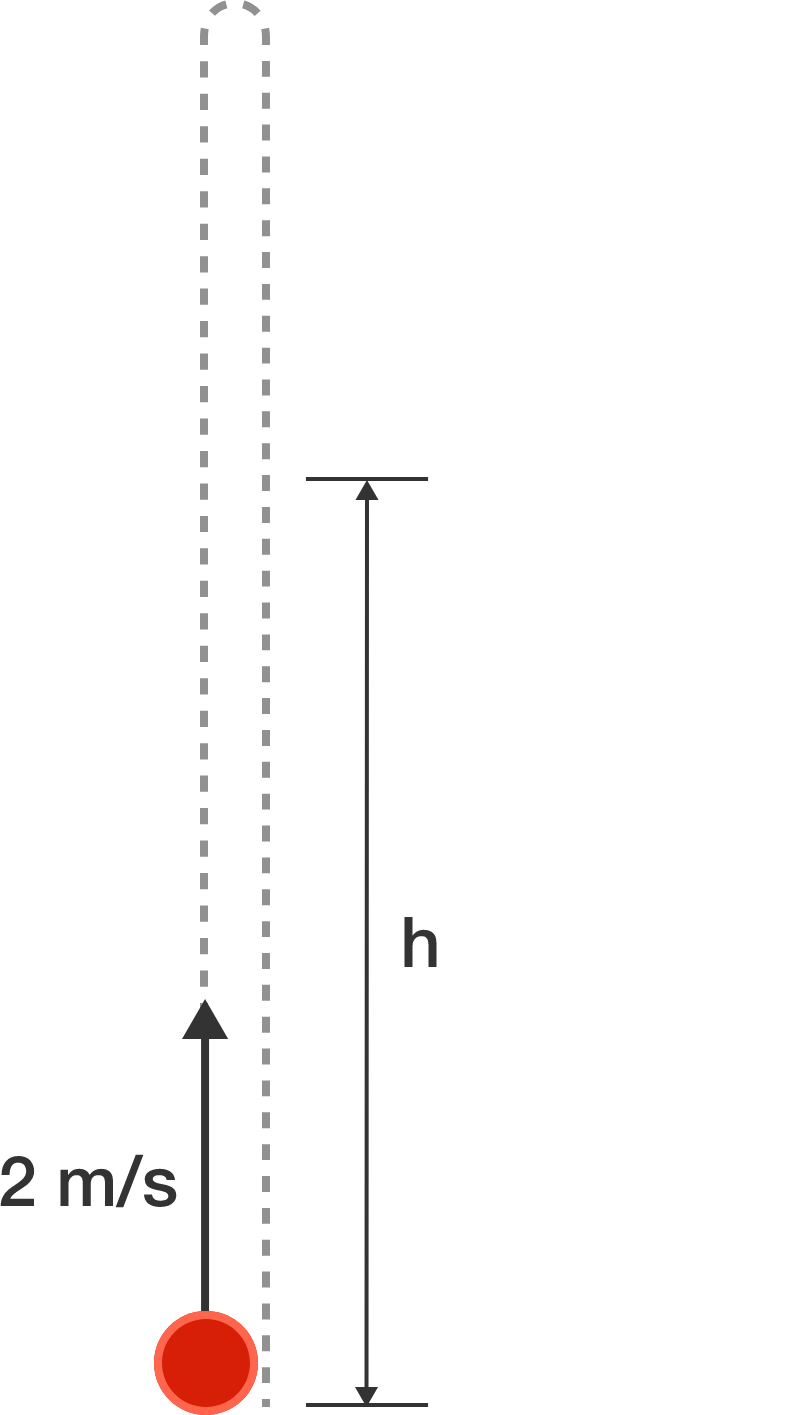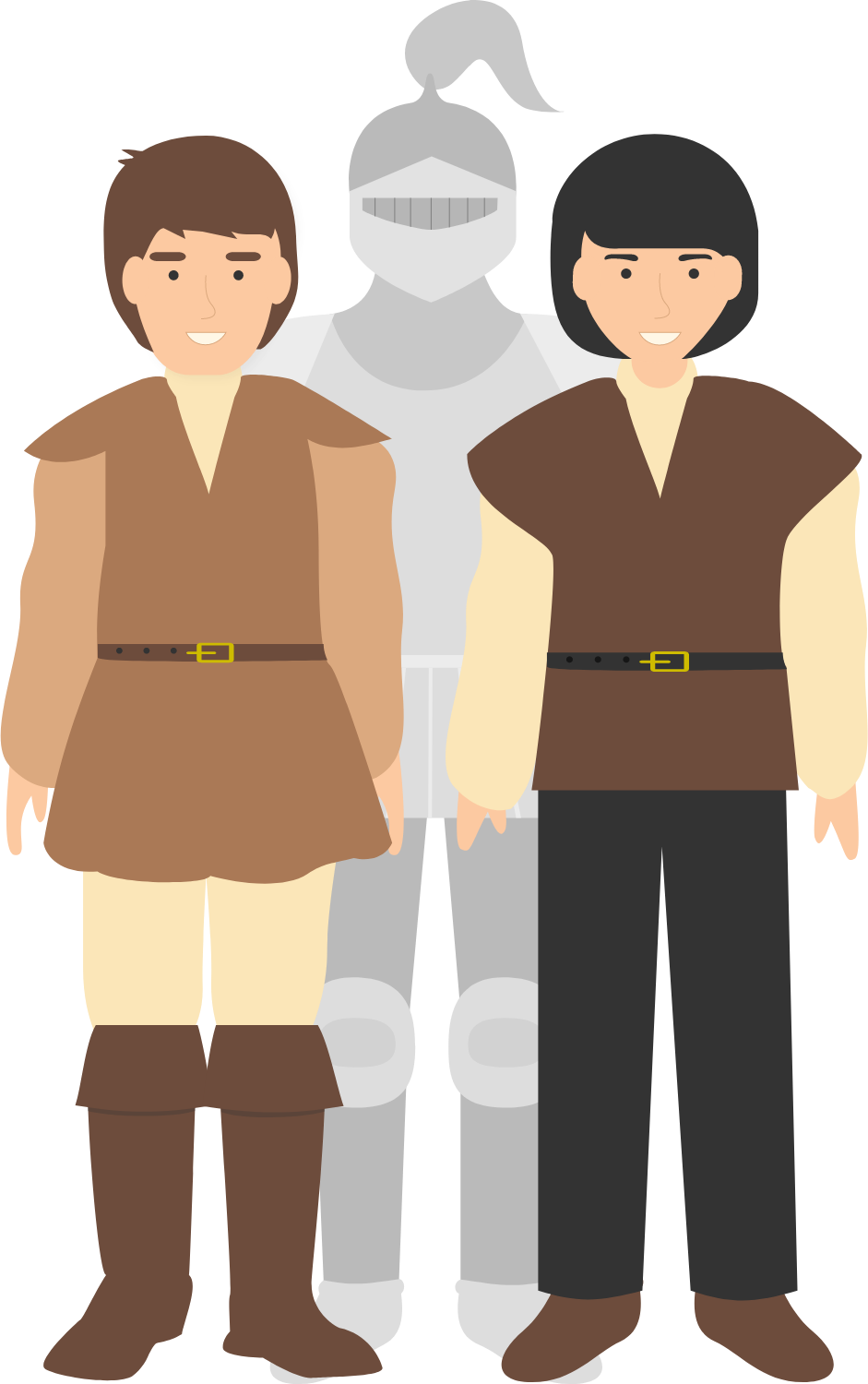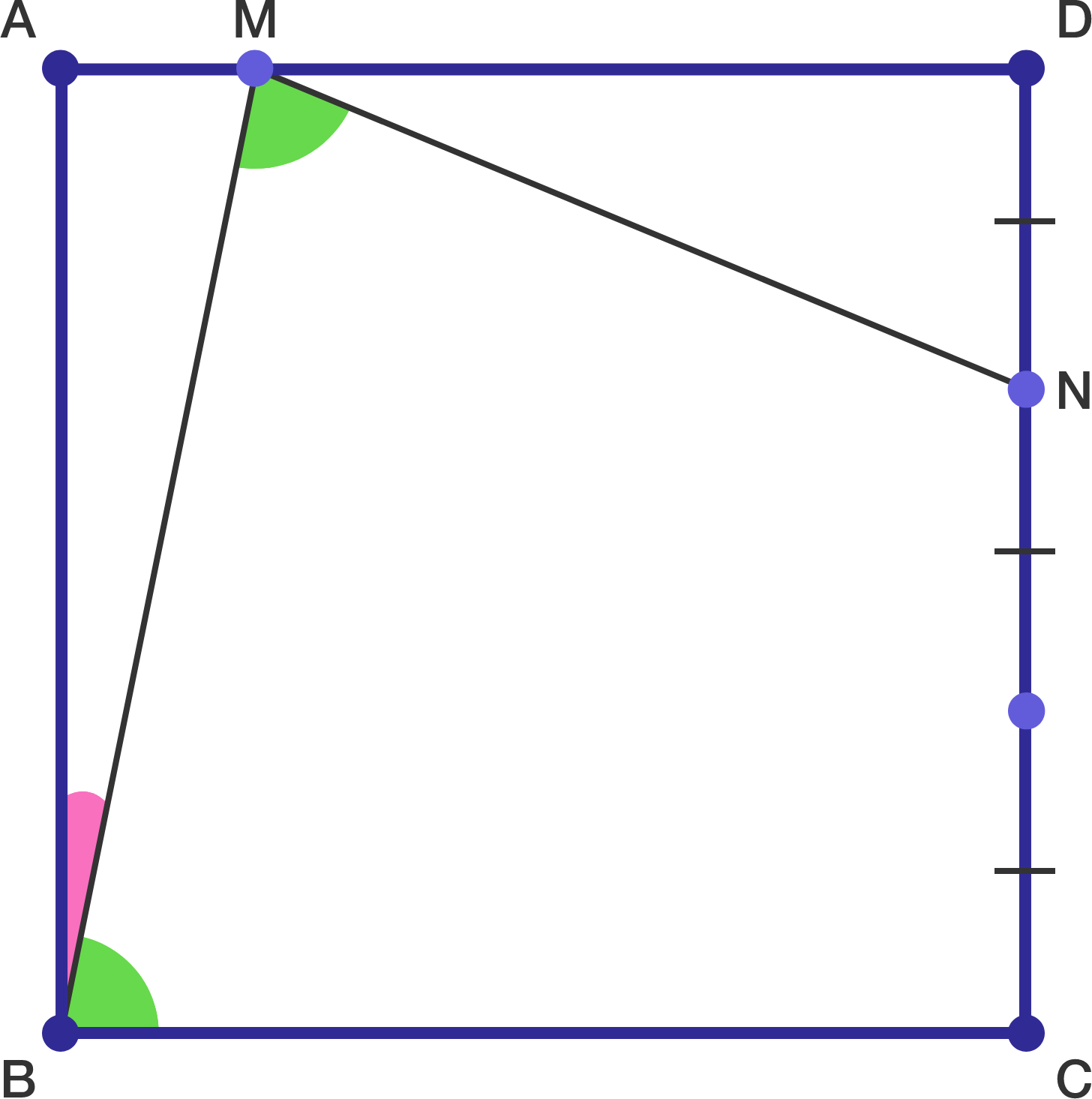# Problems of the Week

Contribute a problem

# 2017-06-05 IntermediateA rocket, initially at rest in deep space, starts its thrusters, which then burn and eject fuel at a uniform rate to provide constant thrust to the rocket.

How does the speed of the rocket vary with time until all of the fuel is exhausted?A ball is thrown vertically upwards with a velocity of $\SI[per-mode=symbol]{2}{\meter\per\second}.$ It is seen at height $h$ twice.

Find the sum of the times, measured in seconds from the moment the ball is thrown, at which it is seen at height $h$.


Details and Assumptions:

• Take $g=\SI[per-mode=symbol]{10}{\meter\per\second\squared}$.
• Neglect air resistance.

Does there exist a power of 2 whose last 4 digits are all identical?Note: $n$ is a positive integer.

You happen to be in a village full of knights (who always tell the truth) and knaves (who always lie), but you can't tell which is which.

You approach two people, Dwight and Dave, one of whom is a knight and the other is a knave. One of them makes the following two statements:

"If I am a knight and he is a knave, then I am Dwight and he is Dave.

But If I am a knave and he is a knight, then I am Dave and he is Dwight."Hmm... knight, knave, Dwight, Dave...

Is the speaker a knight or a knave? Dwight or Dave?


Clarification: As with all knights and knave problems, treat this question as a formal logic question.In the diagram above, $ABCD$ is a square, $NC = 2DN,$ and $\angle NMB = \angle MBC.$

If $MDN$ is not a degenerate triangle (that is, its area is non-zero), what is $\tan \angle ABM?$

×